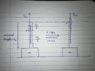# Work: I am having problem determining the elongation in spring

• PhysicsBoi1908

## Homework Statement

A spring has been attached to an object which is kept on the ground. The spring is initially in its natural length: 'l' when a force 'F' is applied on it. The force 'F' slowly pulls the object so it does not attain any acceleration. There's a point 'P' on the line which is positioned l/5 units away from the top of the spring when it was in its natural length. After a few moments, the point 'P' is at the same point where the end of the spring earlier was. We are required to determine the work done by the force. There's no air resistance.
I have also attached an image of the diagram.

## Homework Equations

[/B]
I aim at solving this question via the Work-Energy Theorem for a system of particles.

## The Attempt at a Solution

[/B]
We haven't been given the value for the constant of spring force, so to determine that, I thought about the elongation of the spring, I thought that elongation should be equal to l/5 but my teacher said I was wrong. What is wrong about the elongation that I calculated? I then thought that since when we pull a spring, each portion of the spring gets elongated equally, if the spring gets elongated by 'x', then the elongation at each portion will have to be x/l, but i don't know if I am right or wrong.

#### Attachments

•IMG_2299 2.jpg
40.2 KB · Views: 313

I thought that elongation should be equal to l/5 but my teacher said I was wrong. What is wrong about the elongation that I calculated?
How did you calculate it?

I then thought that since when we pull a spring, each portion of the spring gets elongated equally,
Sounds good to me. So by what factor does the spring stretch?

I assumed that since point P on the spring is going up by l/5, elongation must be l/5 but my sir said that my logic is wrong, he would discuss the solution on Tuesday

I assumed that since point P on the spring is going up by l/5, elongation must be l/5 but my sir said that my logic is wrong, he would discuss the solution on Tuesday
Your logic is wrong but easily fixable. Consider the section of spring from point P to the bottom. By what factor does its length change?

Initially, P was 4l/5 away from the surface, then after elongation, it now is 5l/5 from the surface, I still see an l/5 change or 20% change.

Initially, P was 4l/5 away from the surface, then after elongation, it now is 5l/5 from the surface, I still see an l/5 change or 20% change.
Try again: l/5 is what fraction of 4l/5?

25%

Oh! Now I see it, change in length =(5l/5-4l/5)/4l/5*100=25%

Oh! Now I see it, change in length =(5l/5-4l/5)/4l/5*100=25%
There you go.Thank you very much, sir! I really appreciate how gracefully you led me to the answer without actually giving it out. However, I have one last question, why is it important to consider these values from down and not from the up?

However, I have one last question, why is it important to consider these values from down and not from the up?
Simply because that's the only data given. The length from point P to the top of the spring stretches from l/5 to what? You aren't given that info. (But you can figure it out from what you are given.)